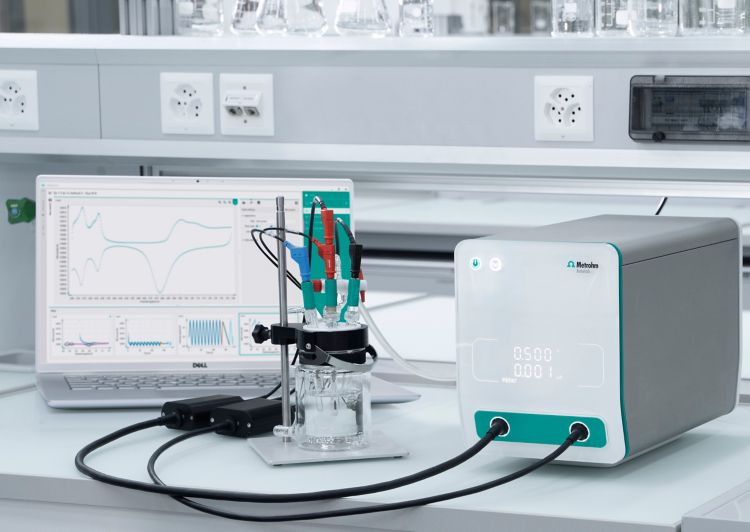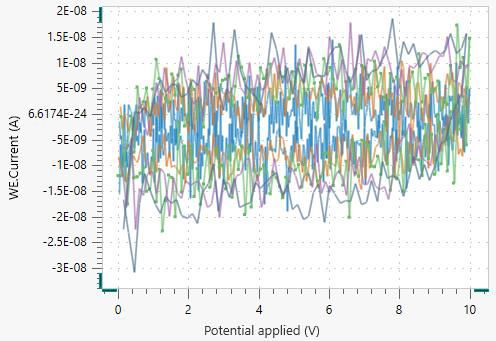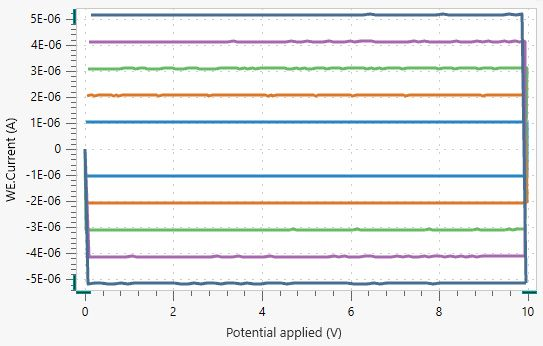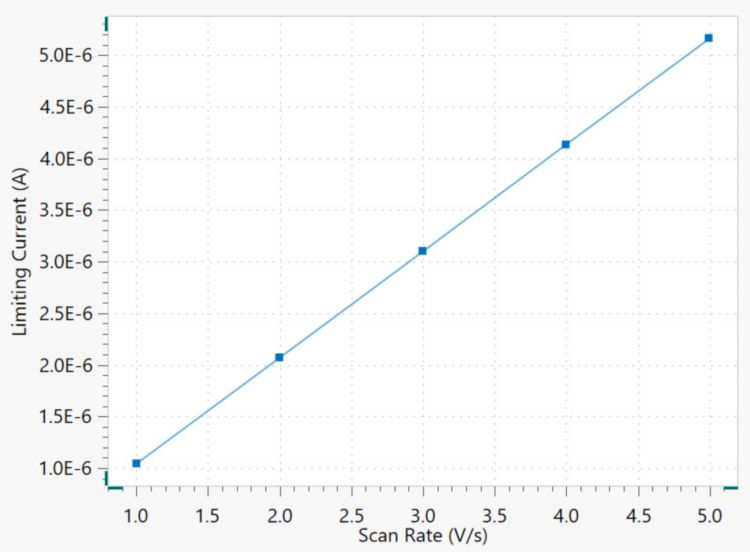You have been redirected to your local version of the requested page

Capacitors are electronic components necessary for the success of the electronics industry. They have also become essential components of both electric and hybrid vehicles.

Electrochemical tests, such as potentiostatic cyclic voltammetry, are used to check the performance of capacitors.

VIONIC powered by INTELLO can perform both staircase and linear cyclic voltammetries (CV). This Application Note gives a comparison between the linear and the staircase potentiostatic cyclic voltammetries and highlights the necessity of using the linear CV to best study the performance of capacitorsThe experiments were carried out with a Metrohm  Autolab VIONIC powered by INTELLO (Figure 1).

The VIONIC instrument is equipped with a linear scan generator as standard, allowing users the possibility to perform linear sweeps and cyclic voltammetries.

The INTELLO procedure was composed using both linear and staircase CVs. For each type of cyclic voltammetry, five scans were performed, with scan rates linearly increasing from 1 V/s to 5 V/s.

The sampling interval of the linear CVs was set at 30 ms, and the step height of the staircase CVs was chosen in order to achieve 30 ms of step duration. In this way, the sampling interval of the linear CV and the step duration of the staircase CV were identical.

In all of the cyclic voltammetries, the potential was scanned from 0 V to 10 V, and back down to 0 V.

For the experiments, a 1 μF capacitor was employed and connected to VIONIC with a two-electrode setup. One terminal of the capacitor was connected to the CE + RE (counter and reference electrode) leads, and the other to the WE + S (working electrode and sense) leads.Figure 2. Cyclic voltammogram resulting from the staircase CV. In light blue are data from the CV at 1 V/s, orange: 2 V/s, green: 3 V/s, purple: 4 V/s, and dark blue: 5 V/s.

In Figure 2, the cyclic voltammograms from the application of the staircase CVs on the capacitor are shown.

It is clearly visible in Figure 2 that the resulting data are arranged in lines resembling a hysteresis, with current values well below the expected values.

With a staircase CV, the measured signal is sampled at the end of the step, in order to record only the faradaic current and to disregard the current given by the charging of the double layer.

However, the current exchanged by a capacitor is non-faradaic. Therefore, it is not possible to measure the current signal of a capacitor with a staircase CV.Figure 3. Cyclic voltammogram resulting from the linear CV. In light blue are data from the CV at 1 V/s, orange: 2 V/s, green: 3 V/s, purple: 4 V/s, and dark blue: 5 V/s.

In Figure 3, the cyclic voltammograms from the linear CVs applied on the capacitor are shown.

All of the cyclic voltammograms resemble the expected shape of the cyclic voltammogram of the capacitor. Also, the value of the current matches the one expected for each scan rate.

In a capacitor, the current i (A) is directly proportional to the scan rate dV/dt (V/s), according to Equation 1.Figure 4. Limiting current versus scan rate of each scan of Figure 3.

The coefficient of proportionality is the capacitance C (F). This is shown in Figure 4, where the limiting current of each linear scan in Figure 3 is plotted against the scan rate.

A linear regression in the data results in a capacitance of 1.03 μF. The plot and the regression analysis were made with NOVA using the INTELLO IN2NOVA button found in the software.

A comparison was performed between linear and staircase cyclic voltammetry on a capacitor, with the results clearly indicating that linear cyclic voltammetry gives trustworthy results when employed to study the performance of capacitors.